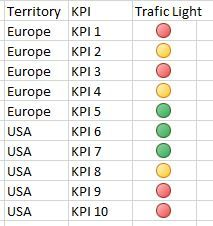Announcements
The way to achieve your own success is the willingness to help somebody else. Go for it!
cancel
Showing results for
Did you mean:Contributor II

## Different traffic light bounds based on dimension value

Dear all,

Could you please give me a hand on this?

I am trying to build a straight table on Qlikview, and one of the Expressions I created is a result in a traffic light format.

The demand is to have different lower and upper bounds based on different regions and KPIs.Since now, Thank you very much

1 Solution

Accepted SolutionsCreator III

you should set the lower bound for each traffic light chart then I think you can use from if statement.

if(territory='USA',1,if(territory='Europe',2)

then you should set the lower bound  for two segment to 0 and 2.

3 RepliesCreator III

you should set the lower bound for each traffic light chart then I think you can use from if statement.

if(territory='USA',1,if(territory='Europe',2)

then you should set the lower bound  for two segment to 0 and 2.Contributor II
Author

Thank you!

Was not exactly like this but open my mind for achieving the solution.

If(Avg({\$<Territory={'A'},KPI={'1'}>}([Result]))>0,If(Avg({\$<Territory={'A'},KPI={'1'}>}([Result]))<0.3,1,2)) &
If(Avg({\$<Territory={'B},KPI={'2'}>}([Result]))>0,If(Avg({\$<Territory={'B'},KPI={'2'}>}([Result]))<0.8,1,2)) &
If(Avg({\$<Territory={'C'},KPI={'3'}>}([Result]))>0,If(Avg({\$<Territory={'C'},KPI={'3'}>}([Result]))<1.3,1,2))

THANKSCreator III
Hi,
if someone is looking at this, there is DEFINITELY an easier way than the above formula.
instead of making a convoluted gauge setting minimum threashhold, simply make your actual expression an If forumla so that the result is either an 0,1 or 2.Community Browser